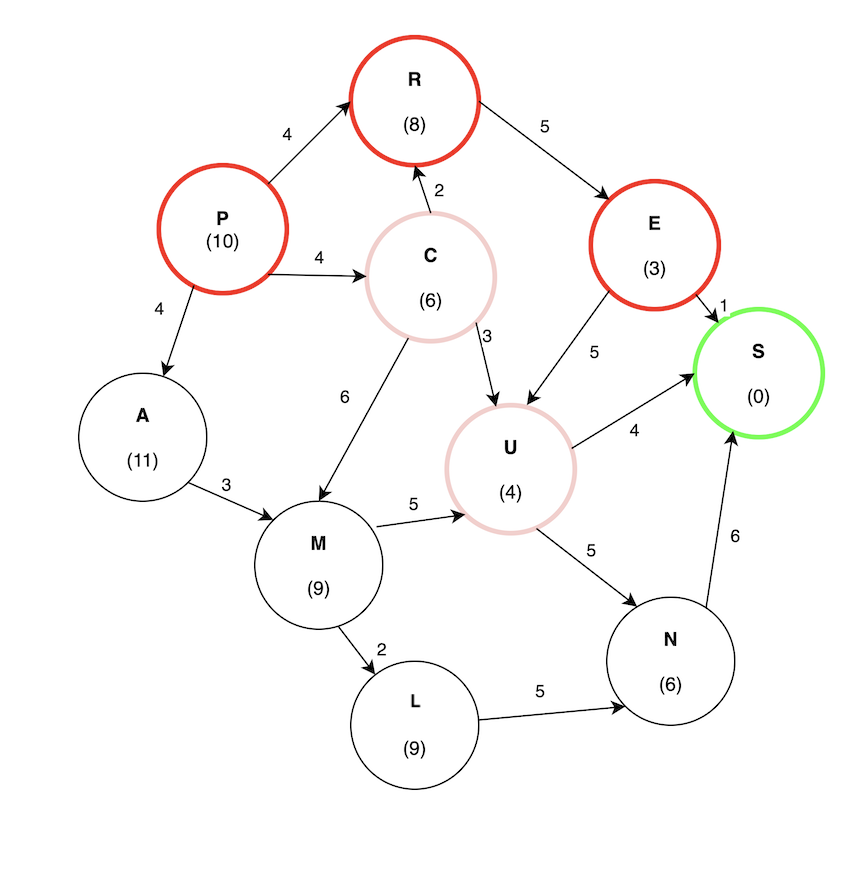# Greedy Best-First Search

Greedy best-first search is an informed search algorithm where the evaluation function is strictly equal to the heuristic function, disregarding the edge weights in a weighted graph because only the heuristic value is considered. In order to search for a goal node it expands the node that is closest to the goal as determined by the heuristic function. This approach assumes that it is likely to lead to a solution quickly. However, the solution from a greedy best-first search may not be optimal since a shorter path may exist.

In this algorithm, search cost is at a minimum since the solution is found without expanding a node that is not on the solution path. This algorithm is minimal, but not complete, since it can lead to a dead end. It’s called “Greedy” because at each step it tries to get as close to the goal as it can.

## Evaluation Function

The evaluation function, f(x), for the greedy best-first search algorithm is the following:

``````f(x) = h(x)
``````

Here, the evaluation function is equal to the heuristic function. Since this search disregards edge weights, finding the lowest-cost path is not guaranteed.

### Heuristic Function

A heuristic function, h(x), evaluates the successive node based on how close it is to the target node. In other words, it chooses the immediate low-cost option. As this is the case, however, it does not necessarily find the shortest path to the goal.

Suppose a bot is trying to move from point A to point B. In greedy best-first search, the bot will choose to move to the position that brings it closest to the goal, disregarding if another position ultimately yields a shorter distance. In the case that there is an obstruction, it will evaluate the previous nodes with the shortest distance to the goal, and continuously choose the node that is closest to the goal.

## The Algorithm

1. Initialize a tree with the root node being the start node in the open list.
2. If the open list is empty, return a failure, otherwise, add the current node to the closed list.
3. Remove the node with the lowest h(x) value from the open list for exploration.
4. If a child node is the target, return a success. Otherwise, if the node has not been in either the open or closed list, add it to the open list for exploration.

## Example

Consider finding the path from P to S in the following graph: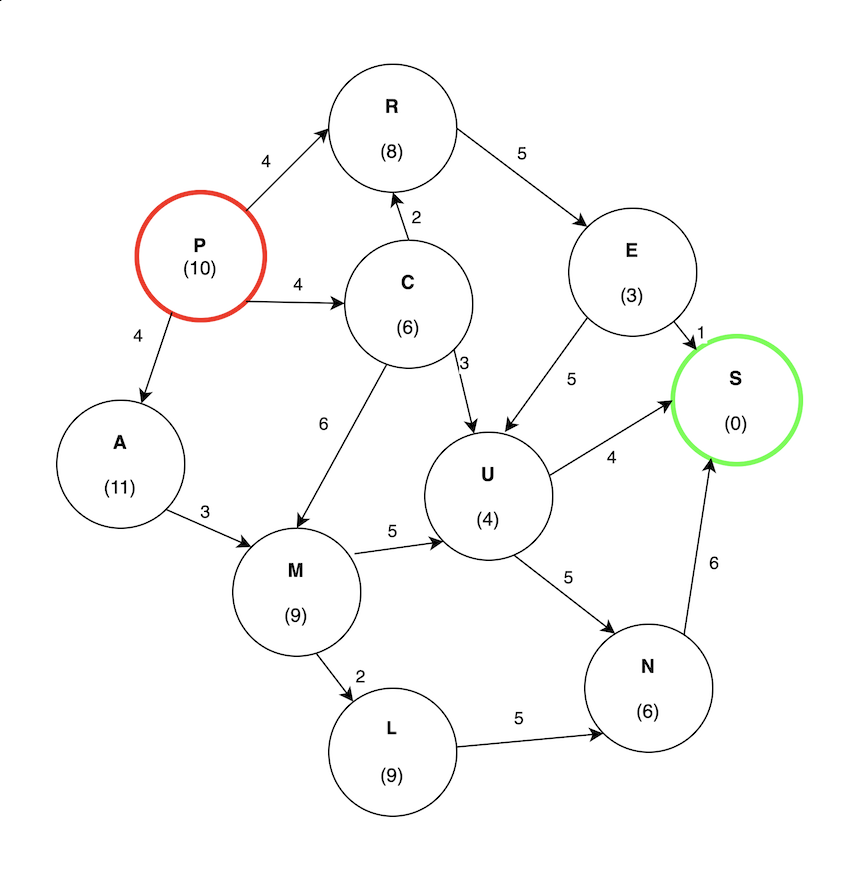In this example, the cost is measured strictly using the heuristic value. In other words, how close it is to the target.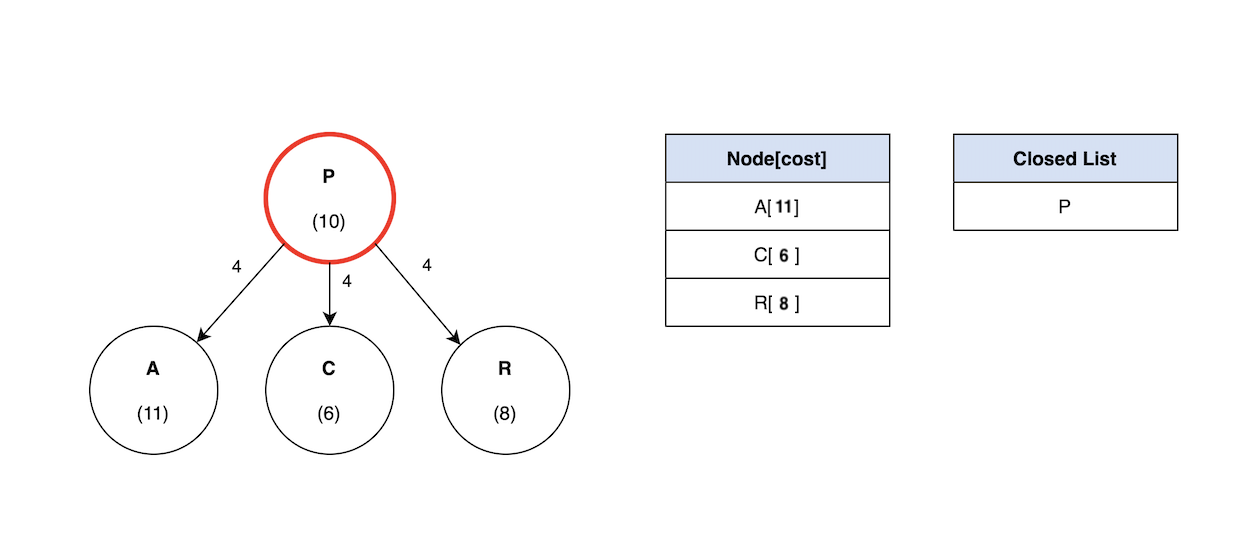C has the lowest cost of 6. Therefore, the search will continue like so: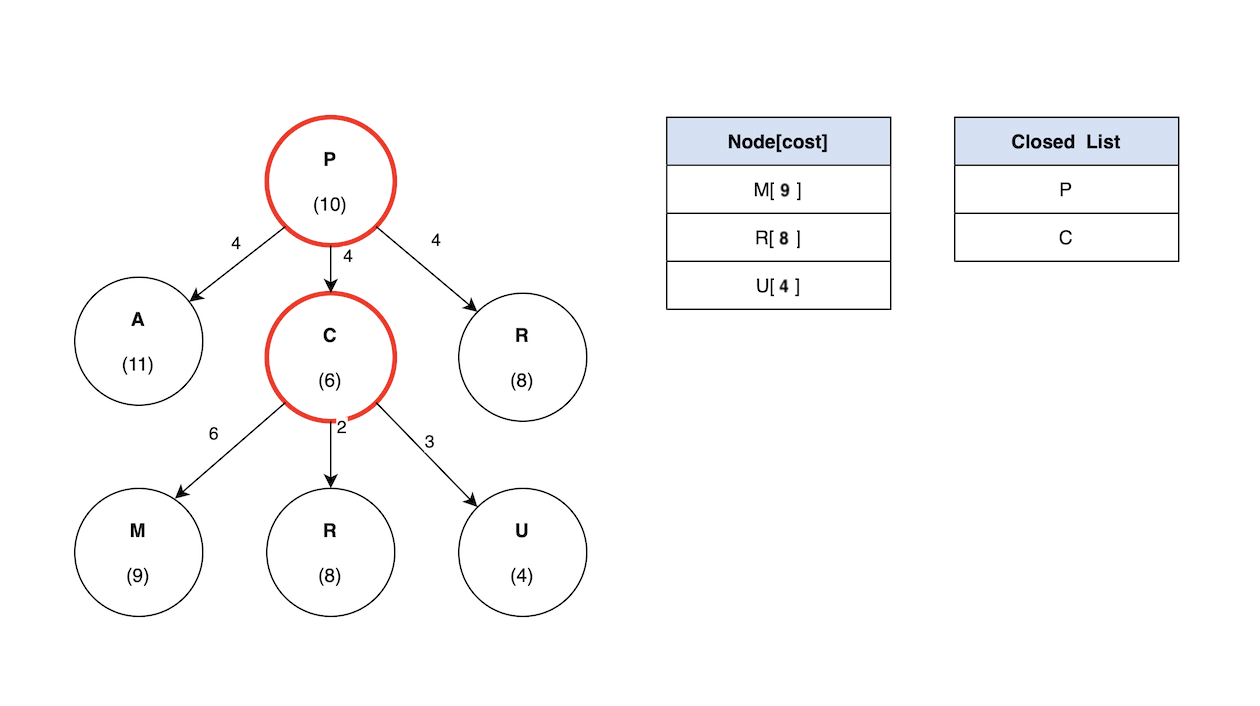U has the lowest cost compared to M and R, so the search will continue by exploring U. Finally, S has a heuristic value of 0 since that is the target node: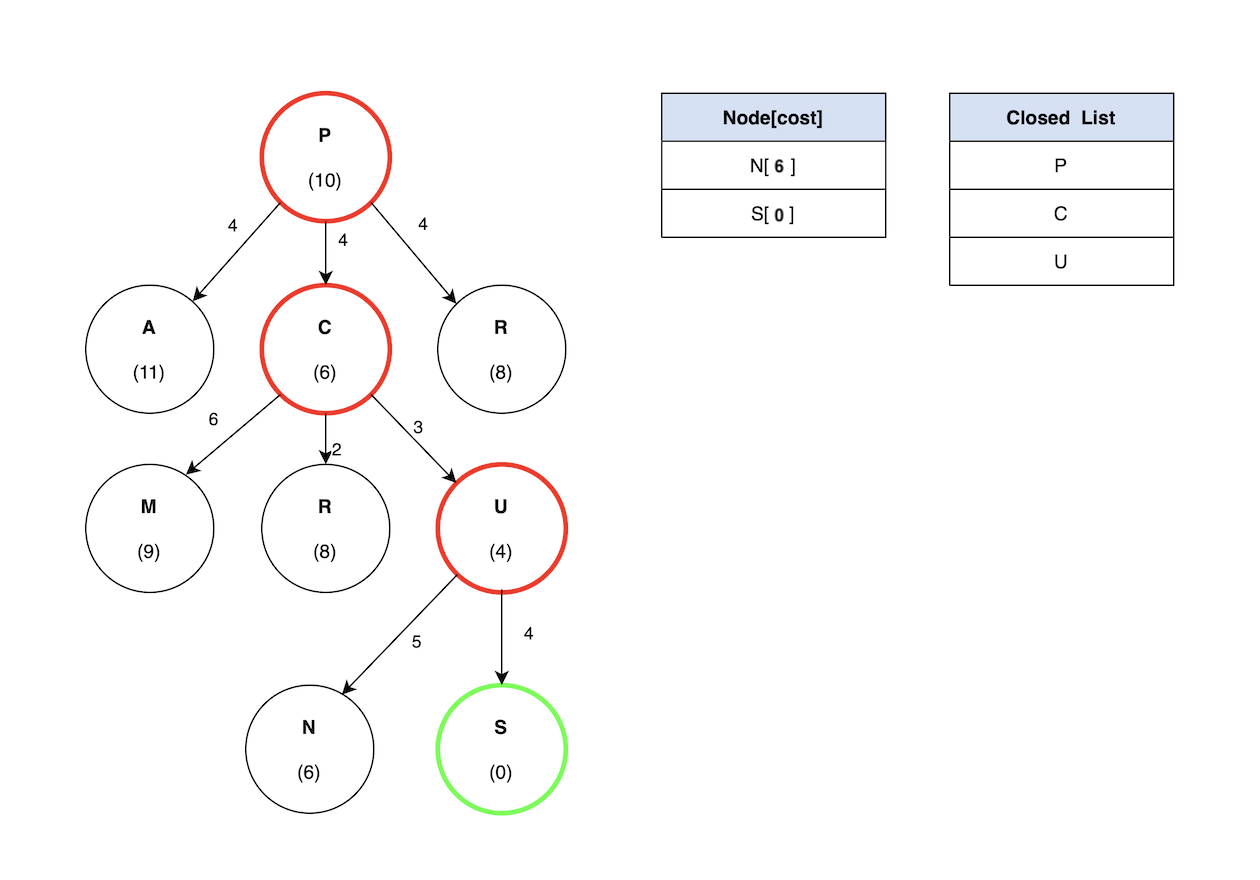The total cost for the path (P -> C -> U -> S) evaluates to 11. The potential problem with a greedy best-first search is revealed by the path (P -> R -> E -> S) having a cost of 10, which is lower than (P -> C -> U -> S). Greedy best-first search ignored this path because it does not consider the edge weights.